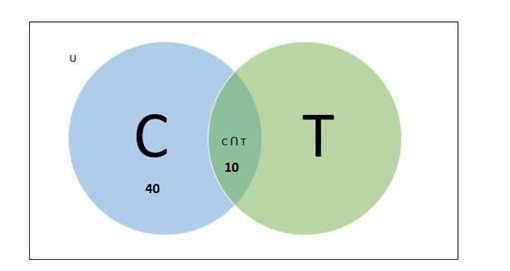# In a group of 65 people, 40 like cricket and 10 like both cricket and tennis.

Question:

In a group of 65 people, 40 like cricket and 10 like both cricket and tennis.

How many like tennis only and not cricket? How many like tennis?

Solution:

Given:

In a group of 65 people:

- 40 people like cricket

- 10 like both cricket and tennis

To Find:

- Number of people like tennis only

- Number of people like tennis

Let us consider,

Number of people who like cricket $=n(C)=40$

Number of people who like tennis $=n(T)$

Number of people who like cricket or tennis $=n(C \cup T)=65$

Number of people who like cricket and tennis both $=n(C \cap T)=10$

Venn diagram:Now,

$n(C \cup T)=n(C)+n(T)-n(C \cap T)$

$65=40+n(T)-10$

$n(T)=65-40+10$

$=35$

Therefore, number of people who like tennis = 35

Now

Number of people who like tennis only $=n(T-C)$

$n(T-C)=n(T)-n(C \cap T)$

$=35-10$

$=25$

Therefore, the number of people who like tennis only = 25